# is no work done, by the gas or the surroundings. Need Help? Sketch a PV diagram...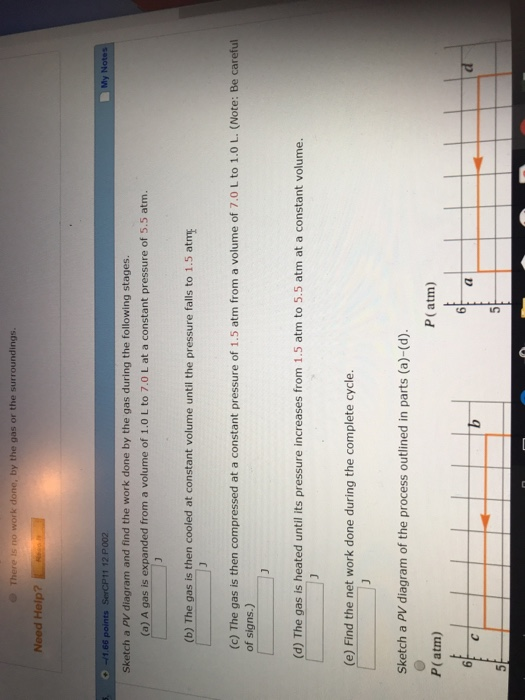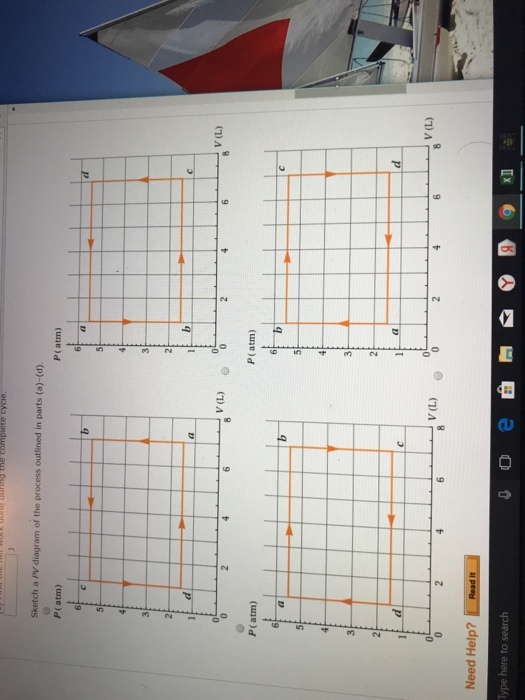is no work done, by the gas or the surroundings. Need Help? Sketch a PV diagram and find the work done by the gas during the following stages. (a) A gas is expanded from a volume of 1.0 L to 7.0 L at a constant pressure of 5.5 atm. (b) The gas is then cooled at constant volume until the pressure falls to 1.5 atm (c) The gas is then compressed at a constant pressure of 1.5 atm from a volume of 7.0 L to 1.0 L. (Note: Be careful of signs.) (d) The gas is heated until its pressure increases from 1.5 atm to 5.5 atm at a constant volume. (e) Find the net work done during the complete cycle. Sketch a PV diagram of the process outlined in parts (a)-(d). P(atm) P(atm) 6 6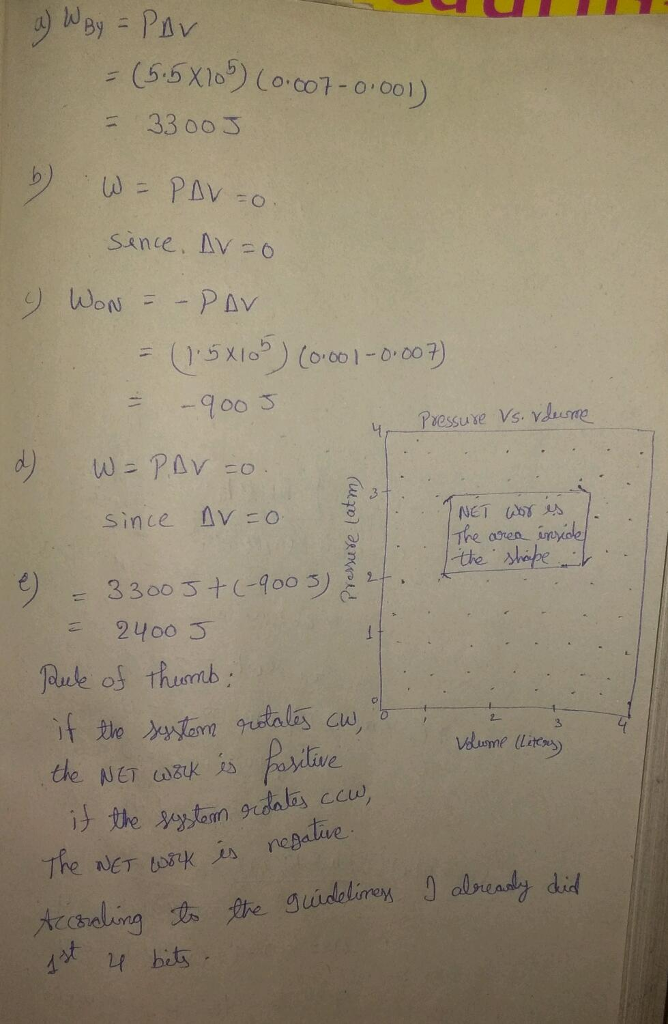#### Earn Coin

Coins can be redeemed for fabulous gifts.

Similar Homework Help Questions
• ### 2) Sketch a PV diagram and find the work done by the gas during the following...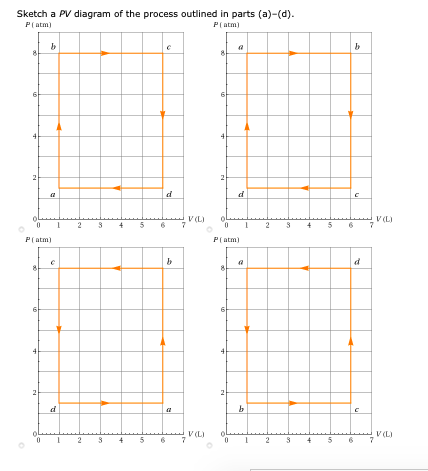2) Sketch a PV diagram and find the work done by the gas during the following stages. (a) A gas is expanded from a volume of 1.0 L to 6.0 L at a constant pressure of 8.0 atm. _ J (b) The gas is then cooled at constant volume until the pressure falls to 1.5 atm. J (c) The gas is then compressed at a constant pressure of 1.5 atm from a volume of 6.0 L to 1.0 L. (Note:...

• ### 1) An ideal gas is enclosed in a cylinder with a movable piston on top of...1) An ideal gas is enclosed in a cylinder with a movable piston on top of it. The piston has a mass of 8,000 g and an area of 5.00 cm2 and is free to slide up and down, keeping the pressure of the gas constant. (a) How much work is done on the gas as the temperature of 0.105 mol of the gas is raised from 10.0°C to 260°C? J b) What does the sign of your answer to...

• ### WORK DONE BY AN IDEAL GAS Ivariant of FSU Physies libl: An ideal monoatomic gas is...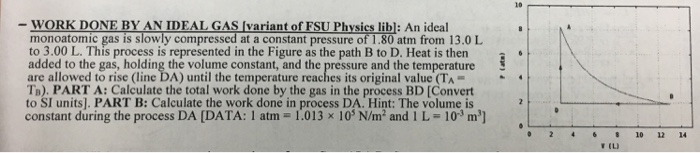WORK DONE BY AN IDEAL GAS Ivariant of FSU Physies libl: An ideal monoatomic gas is slowly compressed at a constant pressure of 1.80 atm from 13.0 L to 3.00 L. This process is represented in the Figure as the path B to D. Heat is then added to the gas, holding the volume constant, and the pressure and the temperature are allowed to rise (line DA) until the temperature reaches its original value (TA- Ta). PART A: Calculate the...

• ### A) Find w12, the work done on the gas as it expands from state 1 to state 2. Express the work done in terms of Po and...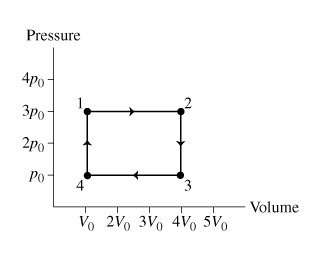A) Find w12, the work done on the gas as it expands from state 1 to state 2. Express the work done in terms of Po and Vo B) Find W23 , the work done on the gas as it cools from state 2 to state 3. Express your answer in terms of Po and Vo. C) Find W34, the work done on the gas as it is compressed from state 3 to state 4. Express your answer in terms...

• ### Step by step please 2. -12 points Tipler6 18.P.050 My Notes Ask Your Teac A gas...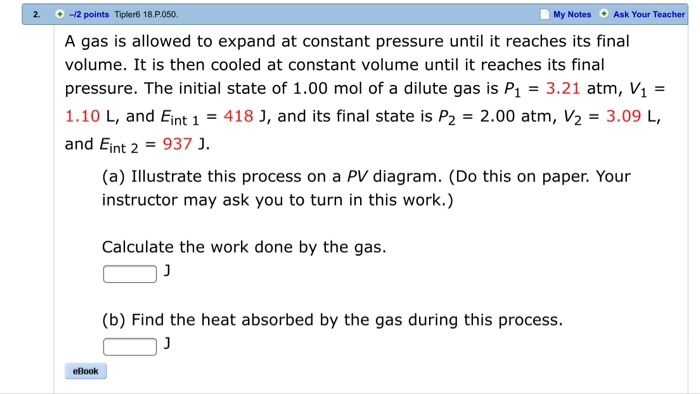Step by step please 2. -12 points Tipler6 18.P.050 My Notes Ask Your Teac A gas is allowed to expand at constant pressure until it reaches its final volume. It is then cooled at constant volume until it reaches its final pressure. The initial state of 1.00 mol of a dilute gas is P1 3.21 atm, V1 - 1.10 L, and Eint 1 = 4183, and its final state is P2 = 2.00 atm, V2 = 3.09 L, and Eint...

• ### work & PV diagram for a gas 2

One mole of a dilute, monoatomic gas initially has a pressure equal to 1.00 atm, and a volume equal to 23.0 L. As the gas is slowly heated, the plot of its state on aPV diagram moves in a straight line to the final state. The gas now has a pressure equal to 3.00 atm, and a volume equal to 71.0 L.(a) Find the work done by the gas.? kJ(b) Find the heat absorbed by the gas.? kJ

• ### A heat engine using a monatomic gas follows the cycle shown in the pV diagram. P11...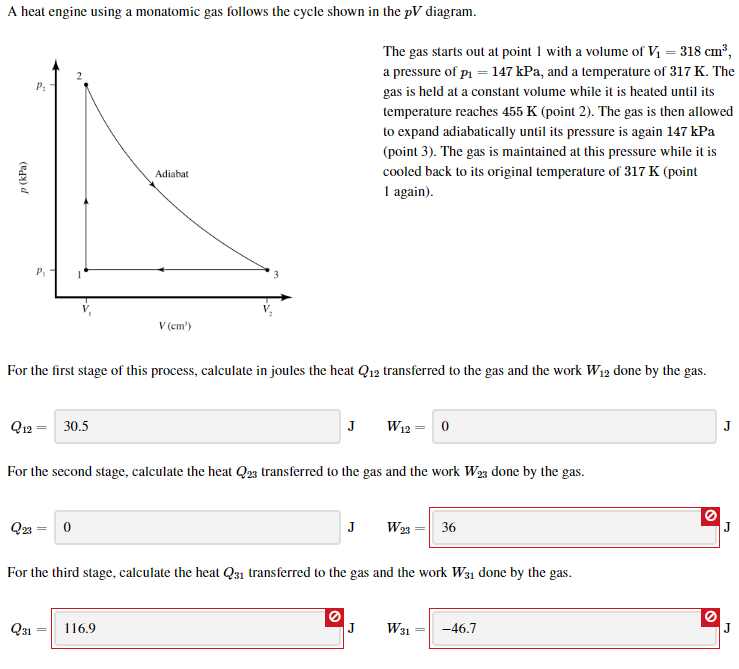A heat engine using a monatomic gas follows the cycle shown in the pV diagram. P11 The gas starts out at point 1 with a volume of V1 = 318 cm", a pressure of p1 = 147 kPa, and a temperature of 317 K. The gas is held at a constant volume while it is heated until its temperature reaches 455 K (point 2). The gas is then allowed to expand adiabatically until its pressure is again 147 kPa (point...

• ### please include a pv diagram 6. One mole of an ideal gas does 3 000 J...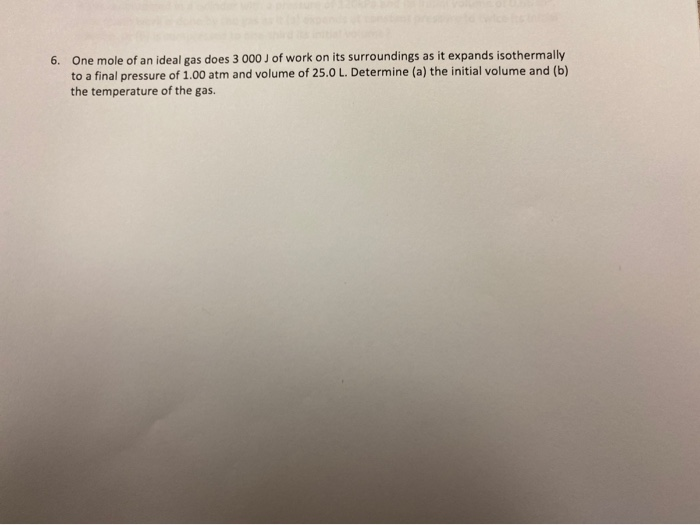please include a pv diagram 6. One mole of an ideal gas does 3 000 J of work on its surroundings as it expands isothermally to a final pressure of 1.00 atm and volume of 25.0 L. Determine (a) the initial volume and (b) the temperature of the gas.

• ### | One mole of an ideal gas does 2750 J of work on its surroundings it...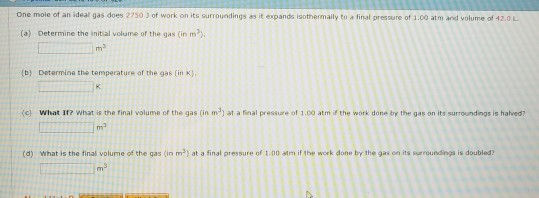| One mole of an ideal gas does 2750 J of work on its surroundings it expands isothermally ta final pressure of 1.00 atm and volume of 42.0 L (a) Determine the initial volume of the gas (in m (b) Determine the temperature of the gas (in K), (c) what lr? what is the final volume of the gas (in m at a final pressure of 1.00 atm if the work done by the gas on its 5urroundings is halved?...

• ### An ideal monatomic gas undergoes changes in pressure and volume, as shown in the pV diagram...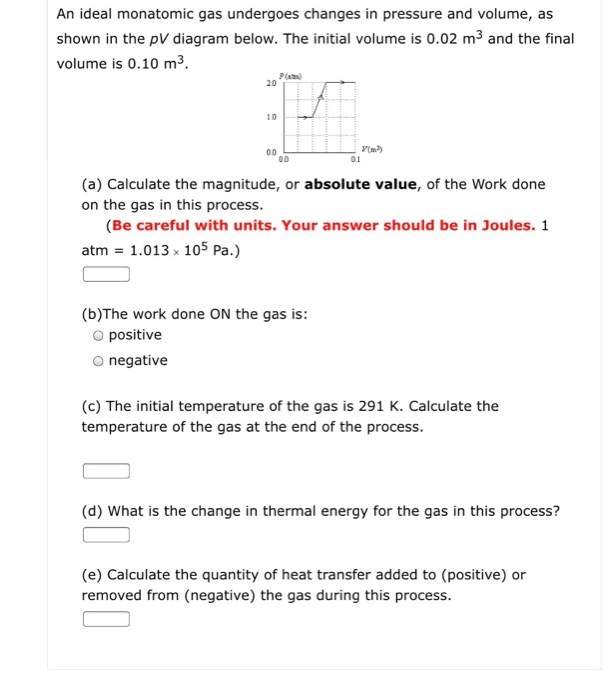An ideal monatomic gas undergoes changes in pressure and volume, as shown in the pV diagram below. The initial volume is 0.02 m3 and the final volume is 0.10 m3 20 10 01 (a) Calculate the magnitude, or absolute value, of the Work done on the gas in this process. (Be careful with units. Your answer should be in Joules. 1 atm 1.013x 105 Pa.) (b)The work done ON the gas is: O positive O negative (c) The initial temperature...